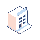• Pricing

# How to declare fields.function in odoo 8 standard I got Error

total_invoiced= fields.function(_invoice_total,string="Total Invoiced",type='float')Hi Libu,

In odoo8 we use Computed fields. For more you can refer : https://www.odoo.com/documentation/8.0/howtos/backend.html#computed-fields-and-default-values

Eg:

name = fields.Char(compute='_compute_name')

def _compute_name(self):

for record in self:

record.name = str(random.randint(1, 1e6))

1Comment1CommentIn odoo 8 functional field is replaced by computed field.

To create a computed field, create a field and set its attribute compute to the name of a method.
The computation method should simply set the value of the field to compute on every record in self.
Here is the simple example.

name = fields.Char(compute='_get_value', string='Name')
value = fields.Char(string='Value')

@api.one
@api.depends('value')
def _get_value(self):
self.name = "Value of name is %s" % self.value

@api.depends('value') decorator specifies the dependency on the 'value' field.
and it is used by the ORM to trigger recomputation of the field.

1Comment